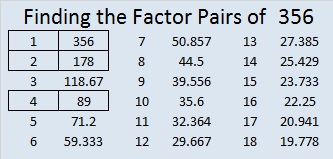# 356 and Level 6

356 is even so it is a composite number. Scroll down past the puzzle to see its factors.Print the puzzles or type the factors on this excel file: 12 Factors 2015-01-12

• 356 is a composite number.
• Prime factorization: 356 = 2 x 2 x 89, which can be written 356 = (2^2) x 89
• The exponents in the prime factorization are 2 and 1. Adding one to each and multiplying we get (2 + 1)(1 + 1) = 3 x 2  = 6. Therefore 356 has exactly 6 factors.
• Factors of 356: 1, 2, 4, 89, 178, 356
• Factor pairs: 356 = 1 x 356, 2 x 178, or 4 x 89
• Taking the factor pair with the largest square number factor, we get √356 = (√4)(√89) = 2√89 ≈ 18.868This site uses Akismet to reduce spam. Learn how your comment data is processed.